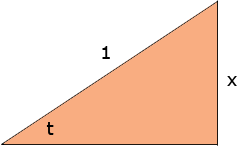Name: scott Who is asking: Student Level of the question: Secondary Question: How to prove arc sin x = arc tan( (x)/√(1-x2)) Hi Scott, Arc sin(x) is the angle whose sine is x. Hence if arc sin(x) = t then sin(t) = x. Think of the number x as x/1 then sin(t) = x/1 Draw a diagram to represent this.Use Pythagoras theorem to find the length of the third side of the triangle. What's the tangent of t? Penny